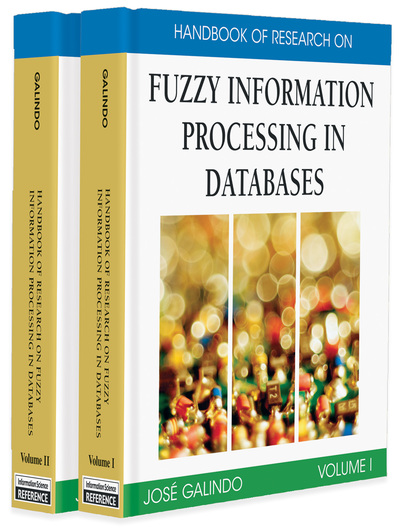# Fuzzy Inclusion Dependencies in Fuzzy Databases

Awadhesh Kumar Sharma (MMM Engg College, Gorakhpur, UP, India), A. Goswami (I.I.T., Kharagpur, India) and D. K. Gupta (I.I.T., Kharagpur, India)
DOI: 10.4018/978-1-59904-853-6.ch026
Available
\$37.50
No Current Special Offers

## Abstract

In this chapter, the concept of fuzzy inclusion dependencies (FIDas) in fuzzy databases is introduced and inference rules on such FIDas are derived. These FIDas may arise while putting efforts to integrate fuzzy relational databases into a fuzzy relational multidatabase. Since the concept of FIDas itself is new, no work has been done as yet for its discovery in fuzzy relational databases. Hence, an algorithm has been proposed for the discovery of FIDas that may exist between two given fuzzy relations stored in one or more fuzzy relational databases. The existence of such an FIDa will indicate that the two relations are relevant for integration during the course of integration of fuzzy databases.

## Key Terms in this Chapter

Partial Fuzzy Inclusion Dependency (FIDa): Let and be (projections on) two fuzzy relations. Let X be a sequence of k distinct fuzzy attribute names from R, and Y be a sequence of k distinct fuzzy attribute names from S, with . Then, a partial fuzzy inclusion dependency is an assertion of the form , such that the fuzzy subset-hood, where is specified in the interval [0,1] and most of the Fuzzy Values under all the attribute names in are Fuzzy Value Equivalent to some Fuzzy Values under respective attribute names in , however, the vice versa may not hold.

Valid FID: A is valid between two relations and if the sets of fuzzy tuples in and satisfy the assertion given by . Otherwise, is called invalid for R and S. In other words, is said to be valid if holds.

Fuzzy Inclusion Dependency (FID): Let and be (projections on) two fuzzy relations. Let X be a sequence of k distinct fuzzy attribute names from R, and Y be a sequence of k distinct fuzzy attribute names from S, with . Then, fuzzy inclusion dependency FID is an assertion of the form , where all the Fuzzy Values under all the attribute names in are Fuzzy Value Equivalent to some Fuzzy Values under respective attribute names in, however, the vice versa may not hold.

Fuzzy Value Equivalent (FVEQ): Fuzzy Value Equivalent (FVEQ): Let A and B be two fuzzy sets with their membership functions and respectively. A fuzzy value is said to be equivalent to some other fuzzy value , for some , where is the set of crisp values that are returned by , where is the inverse of the membership function of fuzzy set A.

Derived FID: A valid FID can be derived from a set of valid FIDs, denoted by ¦, if can be obtained by repeatedly applying the above axioms on some set of FIDs taken from . Similarly, a valid partial inclusion dependency can be derived from a fuzzy set of valid denoted by¦, if can be obtained by repeatedly applying the axioms on some set of FIDs taken from . The membership function of the fuzzy set may be given as follows:, where and, n and m are the cardinality of sets of fuzzy attribute names belonging to fuzzy relations R and S respectively. For example a fuzzy set of valid of arity k may be given as,

Generating Set of FIDa: Consider a fuzzy set of valid partial fuzzy inclusion dependencies:. A generating set of , denoted by, and is a set of valid with the following properties: 1.¦, 2.¦).where the symbol ’’ stands for “fuzzy set-difference”.

Equality of FIDs: Two FIDs and are equal iff there is a sequence () of distinct integers , such that ,. A similar definition holds for the equality of partial fuzzy inclusion dependencies too.

Fuzzy Relational Database of Type-2: A type-2 fuzzy relation r is a fuzzy subset of D, where must satisfy the condition where .

Fuzzy Relational Database of Type-1: In type-1 fuzzy relations, may be a classical subset or a fuzzy subset of . Let the membership function of be denoted by , for . Then from the definition of Cartesian product of fuzzy sets, is a fuzzy subset of . Hence a type-1 fuzzy relation r is also a fuzzy subset of with membership function.

a-Cut: Given a fuzzy set defined on and any number, the -cut , and the strong -cut, , are the crisp sets ,

## Complete Chapter List

Search this Book:
Reset